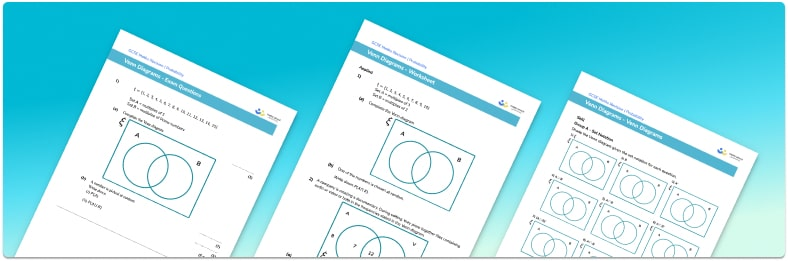# Venn Diagram Worksheet• Section 1 of the Venn diagram worksheet contains 36 skills-based Venn diagram  questions, in 3 groups to support differentiation
• Section 2 contains 4 applied Venn diagram questions with a mix of worded problems and deeper problem solving questions
• Section 3 contains 4 foundation and higher level GCSE exam style Venn diagram questions
• Answers and a mark scheme for all Venn diagram questions are provided
• Questions follow variation theory with plenty of opportunities for students to work independently at their own level
• All questions created by fully qualified expert secondary maths teachers
• Suitable for GCSE maths revision for AQA, OCR and Edexcel exam boards

• This field is for validation purposes and should be left unchanged.

You can unsubscribe at any time (each email we send will contain an easy way to unsubscribe). To find out more about how we use your data, see our privacy policy.

### Venn diagram at a glance

A Venn diagram uses overlapping circles to show the relationship between two or three sets of objects.

The symbol ξ denotes the universal set. The universal set will usually contain a two or three circle Venn diagram. The intersection of sets within a Venn diagram is where sets overlap; A∩B contains every object that is in A and B. The union of sets describes the objects within multiple regions on a Venn diagram; A∪B contains every object that is in A or B. The complement to a set is every object that is not within the set; A’ is every object not in A. Students study set notation to describe shaded regions on a Venn diagram.

E.g. Let ξ={1,2,3,4,5}, A={Even numbers}, and B={Prime numbers}. Then:

• A∩B={2}, the only even prime number;
• A∪B={2,3,4,5}, even numbers or prime numbers;
• A’={1,3,5}, not an even number (odd numbers).

Venn diagrams are useful as they clearly show the relationship between two sets of data and they can be used to help us work out probabilities.

Another diagram for organising sets of data is a Carroll diagram.

Looking forward, students can then progress to additional probability worksheets, for example a probability tree diagram worksheet or

For more teaching and learning support on probability our GCSE maths lessons provide step by step support for all GCSE maths concepts.

## Do you have KS4 students who need more focused attention to succeed at GCSE?There will be students in your class who require individual attention to help them succeed in their maths GCSEs. In a class of 30, it’s not always easy to provide.

Help your students feel confident with exam-style questions and the strategies they’ll need to answer them correctly with our dedicated GCSE maths revision programme.

Lessons are selected to provide support where each student needs it most, and specially-trained GCSE maths tutors adapt the pitch and pace of each lesson. This ensures a personalised revision programme that raises grades and boosts confidence.

Find out more